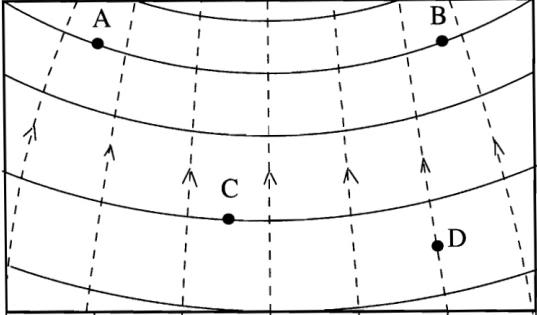# Electric Fields directions

dimpledur

## Homework Statement

I just have a couple of questions concerning electric field.Is the electric field in the direction of path zero at every point from a to b in a straight line?
What about along the equipotential line joining a-b?
Is the electric field in the direction perpendicular to the path zero in the straight line joining a-b?
What about the equipotential line joining a-b?

## The Attempt at a Solution

The main thing that is confusing me is what is this "path" the question refers to? Would that be the path going from one equipotential line to the next?

Homework Helper
hi dimpledur!The main thing that is confusing me is what is this "path" the question refers to?

i think it means the straight line ABdimpledur
I'm really not understanding the wording. Wouldnt the electric field only be zero if it were tangent to the equipotential lines?

Homework Helper
I'm really not understanding the wording. Wouldnt the electric field only be zero if it were tangent to the equipotential lines?

that's right!so the answers are … ?

dimpledur
Perhaps someone could just tell me whether or not I have a grasp on this conceptually?

Is the electric field in the direction of path zero at every point from a to b in a straight line?
I would suggest that if you were to make a line segment from a to b, the only position along that line where the electric field would be zero, is in the middle where the line is perpendicular to the line of force.

What about along the equipotential line joining a-b?
The electric field would be zero along this line..

Is the electric field in the direction perpendicular to the path zero in the straight line joining a-b?
I would say no. It is never zero along this line.

What about the equipotential line joining a-b?
Definitely never zero.

Homework Helper
yes, all four answers are finedimpledur
Thank you. The questions are all multiple choice yes/no answers, however, I figured I should double check since it appeared wordy to me.

dimpledur
My answers would not be affected if one were to change "electric field" to "average electric field", correct? For example, the average electric field in the direction of the path is zero on the path, from point A to point B along a straight line. Yes?

Homework Helper
i suppose so …

but that doesn't seem a likely interpretationdimpledur
Don't get me wrong, I don't like these questions. However, that was another question. Is the electric field in the direction of the path zero on the path from the point A to B along a straight line?

What kind of questions are these?

they do seem very badly worded